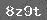# 第三单元达标自测题

（一）填空题。

1．求几个（    ）加数的和，用乘法计算比较简便。

2．①4× 5=  20读作（    ）。

6×3= 18读作（    ）。

1×2= 2读作（    ）。

4×1= 4读作（    ）。

3．下面的乘法算式各表示什么意思：

3×5表示（    ）个（    ）连加。

6×2表示（    ）个（    ）连加。

4×3中，被乘数是（    ），乘数是（    ），相同的加数是（    ），相同加数的个数是（    ）。

（二）读一句口诀写出两个乘法算式。（三）把得数相同的算式用线连起来。

eq /x(5+5)        eq /x(5+5+5)

eq /x(6+6+6+6)    eq /x(6+6+6+6+6)

eq /x(6×5)       eq /x(6×4)

eq /x(5×3)       eq /x(5×2)

eq /x(4×3)       eq /x(3×4)

（四）计算题。

3×4+40     2×5+0

6 × 6- 30    5 × 4- 20

8+5×3      6×2+18

5×5+6      3×4+12

6 ×3+8     35-1×5

（五）列式计算。

135是多少？

263相加得多少？

34乘以5得多少？

4．被乘数是6，乘数是4，积是多少？

5．乘数是5，被乘数是3，积是多少？

（六）先把有关的条件和问题连起来，再计算。

1eq /x(一支6分钱，买5支铅笔，)          eq /x(还剩下几本？)

2eq /x(一个笼子关5只3

  下一页

### 相关推荐

 留言与评论（共有 0 条评论）

 验证码：